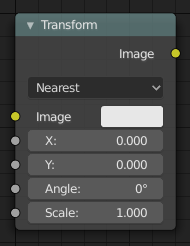# Transform Node¶Transform Node.

This node combines the functionality of three other nodes: Scale, translate, and rotate nodes.

## Inputs¶

Image

Standard image input.

X, Y

Used to move the input image horizontally and vertically.

Angle

Used to rotate an image around its center. Positive values rotate counter-clockwise and negative ones clockwise.

Scale

Used to resize the image. The scaling is relative, meaning a value of 0.5 gives half the size and a value of 2.0 gives twice the size of the original image.

## Properties¶

Filter

Interpolation Methods.

Nearest

No interpolation, uses nearest neighboring pixel.

Bilinear